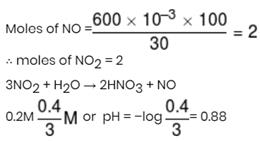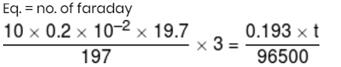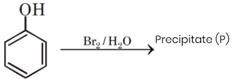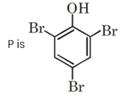Courses

# JEE Main Chemistry Mock - 4

## 25 Questions MCQ Test Mock Test Series for JEE Main & Advanced 2021 | JEE Main Chemistry Mock - 4

Description
This mock test of JEE Main Chemistry Mock - 4 for JEE helps you for every JEE entrance exam. This contains 25 Multiple Choice Questions for JEE JEE Main Chemistry Mock - 4 (mcq) to study with solutions a complete question bank. The solved questions answers in this JEE Main Chemistry Mock - 4 quiz give you a good mix of easy questions and tough questions. JEE students definitely take this JEE Main Chemistry Mock - 4 exercise for a better result in the exam. You can find other JEE Main Chemistry Mock - 4 extra questions, long questions & short questions for JEE on EduRev as well by searching above.
QUESTION: 1

### Phenol can be distinguished from ethyl alcohol by all reagents except

Solution: Phenol reacts with NaOH, doesn't give Bromine water test but alchohol give Bromine water test and don't react with NaOH as pKa of alchohol is more than that of H2O but Na reacts with both phenol and alchohol so Na doesn't distinguish phenol and alchohol. hence D is correct
QUESTION: 2

Solution:
QUESTION: 3

### If R is the Rydberg constant for hydrogen, then wave-number of the second line in the Lyman series is

Solution:

Wave no = 1/λ =R[1/n12 -1/n22]=R[1/12 -1/22]=3R/4.(For Lyman series n1=1)

QUESTION: 4

The ratio of specific charge of a proton and an α-particle is

Solution:
QUESTION: 5
Electrophiles are
Solution:
QUESTION: 6

which of the following hybridisation occurs in the formation of methane molecule?

Solution:
QUESTION: 7
Acetic acid is produced with the help of
Solution:
QUESTION: 8
Structurally, which of the following are similar ions?
Solution:
QUESTION: 9
The molecule having bond order 3 is
Solution:
QUESTION: 10
Tautomerism is exhibited by
Solution:
QUESTION: 11

Which of the following has highest molar conductivity?

Solution:
QUESTION: 12
Which of the following is not a π bonded complex?
Solution:
QUESTION: 13

An aqueous solution of CoCl₂ on addition of excess of concentrated HCl, turns blue due to the formation of

Solution:
QUESTION: 14
Noble gases are
Solution:
QUESTION: 15
Which of the following will act as Lewis base, nucleophilic reagent as well as ligand in complex compound ?
Solution:
QUESTION: 16

Which of the following exhibit geometrical isomerism?

Solution:
QUESTION: 17
Which of the following is obtained when chloral is boiled with NaOH?
Solution:
QUESTION: 18
Effective nuclear charge in case of an ion is equal to-
Solution:
QUESTION: 19
Bakelite is prepared from
Solution:
QUESTION: 20
K, Ca and Li metals may be arranged in the decreasing order of their standard electrode potentials as
Solution:
*Answer can only contain numeric values
QUESTION: 21

Nitric oxide is formed in the combustion of fuel. An internal combustion engine produces 600 ppm (600 mg L–1) of NO. 100 L of air containing the produced NO was oxidised to NO2. The NO2 formed was dissolved in 10 L of water. The pH of the resulting solution is (log 2 = 0.30, log 3 = 0.48)

Solution:*Answer can only contain numeric values
QUESTION: 22

A thin circular-disk earrings 10.0 cm in diameter is plated with a coating of gold 0.20 mm thick from an Au3+ bath. How many days (approx.) does it take to deposit the gold on one side of one earring if the current is 0.193 ampere.
(Au = 197, density of Au = 19.7 g/cm3, 1 F = 96500 C, 86400 = 27500 p)

Solution:*Answer can only contain numeric values
QUESTION: 23

Consider the following orders given below.
(i) S > Se > Te > O (Electron Affinity)
(ii) N–3 > O2– > F > Na+ > Mg2+ (Ionic Size)
(iii) Fe2+ > Fe3+ > Fe4+ (Ionization Enthalpy)
(iv) H < B < P < C < Cl (Electronegativity)
(v) Na+ < Mg2+ < Al3+ (Extent of hydration)
(vi) Li > Na > K > Rb > Cs (Reducing Nature)
(vii) LiF(s) > LiCl(s) > LiBr(s) (Lattice Energy)
If x = number of correct orders
y = number of incorrect orders
then the value of (1 + y/x) is.

Solution:
*Answer can only contain numeric values
QUESTION: 24

Among the following oxides
Cr2O3, V2O5, FeO, Mn2O7, ZnO, Cu2O, MnO2, CrO3, NiO
If x = number of basic oxides
y = number of acidic oxides
z = number of amphoteric oxides
then the value of (x + y)/z is

Solution:
*Answer can only contain numeric values
QUESTION: 25How many hydrogen atom(s) is/are present in precipitate (P)?

Solution: